OpenGL: 如何利用 Shader 实现 RGBA 到 NV21 图像格式转换？（全网首次开源）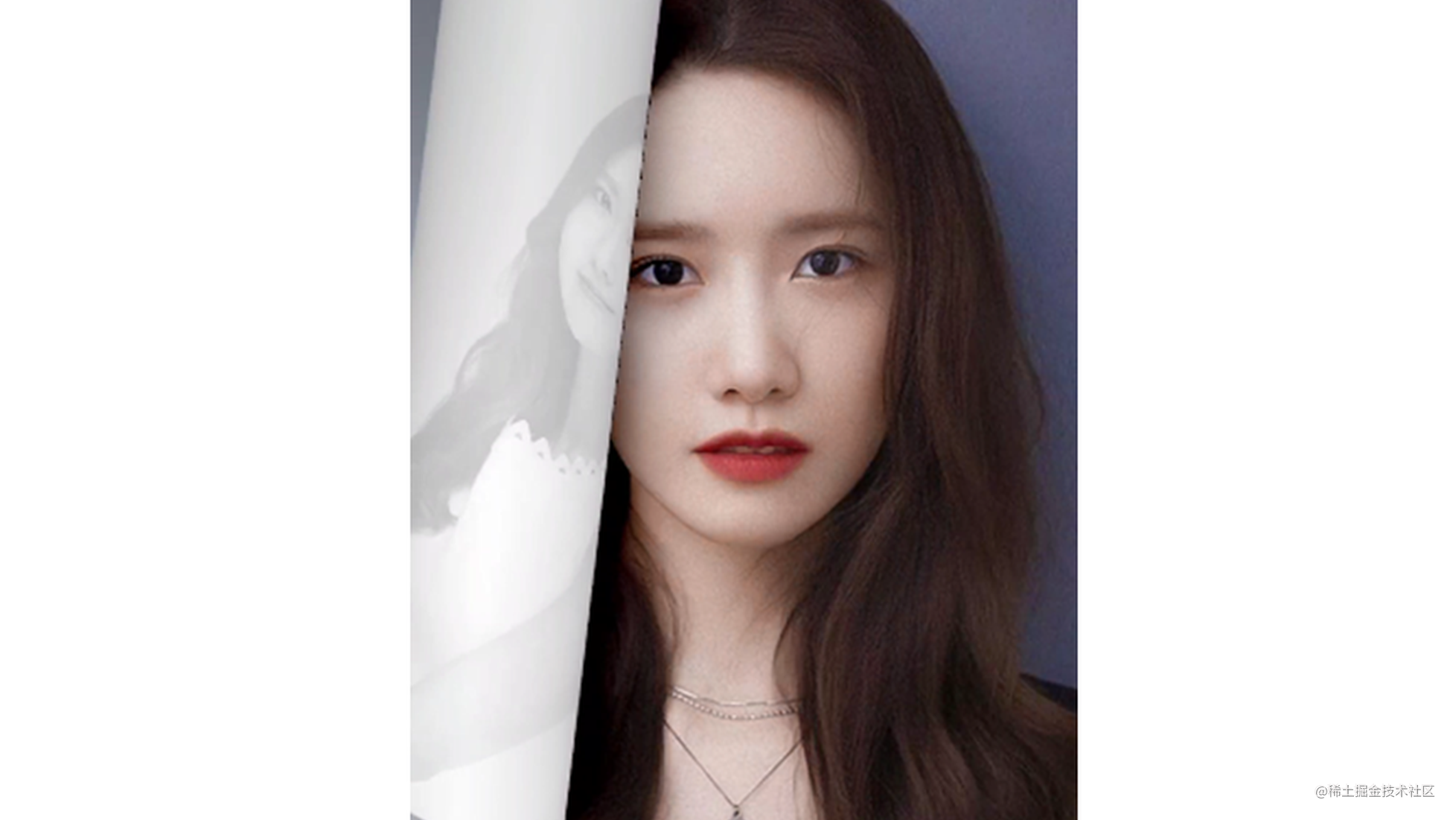10bit YUV（P010）的存储结构和处理

Shader 实现 RGBA 转 NV21 原理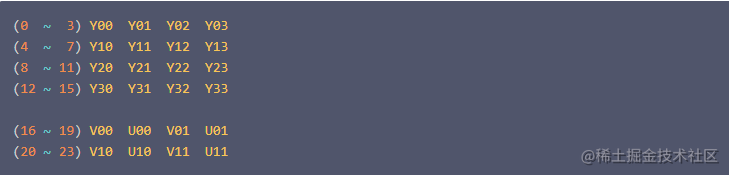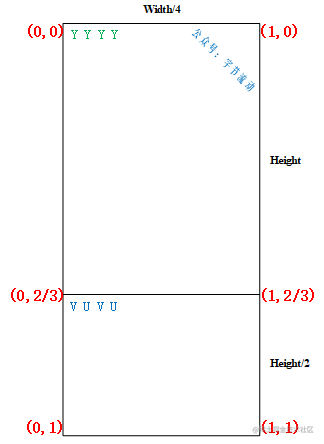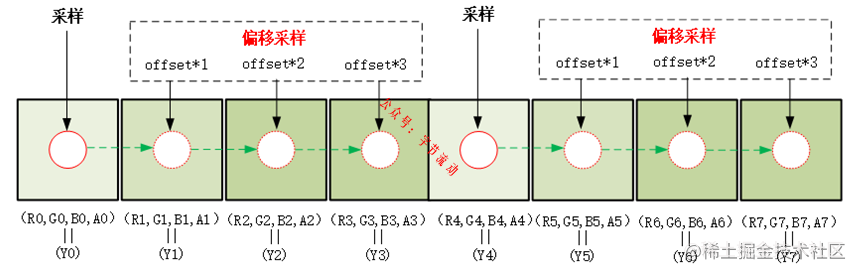同样，生成 VU plane 的图像也需要进行 3 次额外的偏移采样。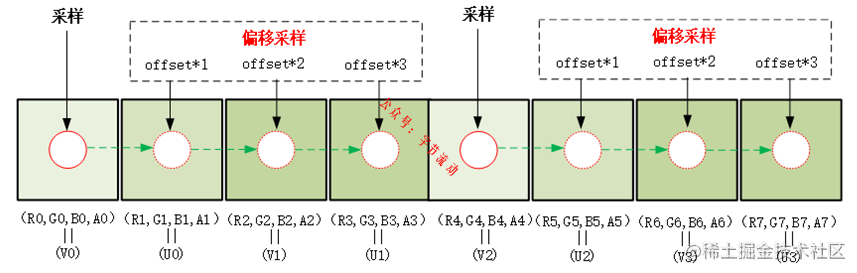offset 需要设置为一个像素归一化之后的值：1.0/width, 按照原理图，为了便于理解，这里将采样过程简化为以 4 个像素为单位进行，在纹理坐标 y < (2/3) 范围，一次采样（加三次偏移采样）4 个 RGBA 像素（R,G,B,A）生成 1 个（Y0,Y1,Y2,Y3），整个范围采样结束时填充好 width*height 大小的缓冲区；

glReadPixels(0, 0, width / 4, height * 1.5, GL_RGBA, GL_UNSIGNED_BYTE, pBuffer);

代码实现

bool RGB2NV21Sample::CreateFrameBufferObj()
{
// 创建并初始化 FBO 纹理
glGenTextures(1, &m_FboTextureId);
glBindTexture(GL_TEXTURE_2D, m_FboTextureId);
glTexParameterf(GL_TEXTURE_2D, GL_TEXTURE_WRAP_S, GL_CLAMP_TO_EDGE);
glTexParameterf(GL_TEXTURE_2D, GL_TEXTURE_WRAP_T, GL_CLAMP_TO_EDGE);
glTexParameteri(GL_TEXTURE_2D, GL_TEXTURE_MIN_FILTER, GL_LINEAR);
glTexParameteri(GL_TEXTURE_2D, GL_TEXTURE_MAG_FILTER, GL_LINEAR);
glBindTexture(GL_TEXTURE_2D, GL_NONE);

// 创建并初始化 FBO
glGenFramebuffers(1, &m_FboId);
glBindFramebuffer(GL_FRAMEBUFFER, m_FboId);
glBindTexture(GL_TEXTURE_2D, m_FboTextureId);
glFramebufferTexture2D(GL_FRAMEBUFFER, GL_COLOR_ATTACHMENT0, GL_TEXTURE_2D, m_FboTextureId, 0);
glTexImage2D(GL_TEXTURE_2D, 0, GL_RGBA, m_RenderImage.width / 4, m_RenderImage.height * 1.5, 0, GL_RGBA, GL_UNSIGNED_BYTE, nullptr);
if (glCheckFramebufferStatus(GL_FRAMEBUFFER)!= GL_FRAMEBUFFER_COMPLETE) {
LOGCATE("RGB2NV21Sample::CreateFrameBufferObj glCheckFramebufferStatus status != GL_FRAMEBUFFER_COMPLETE");
return false;
}
glBindTexture(GL_TEXTURE_2D, GL_NONE);
glBindFramebuffer(GL_FRAMEBUFFER, GL_NONE);
return true;

}

#version 300 es
precision mediump float;
in vec2 v_texCoord;
layout(location = 0) out vec4 outColor;
uniform sampler2D s_TextureMap;
uniform float u_Offset;  //偏移量 1.0/width
//Y =  0.299R + 0.587G + 0.114B
//U = -0.147R - 0.289G + 0.436B
//V =  0.615R - 0.515G - 0.100B
const vec3 COEF_Y = vec3( 0.299,  0.587,  0.114);
const vec3 COEF_U = vec3(-0.147, -0.289,  0.436);
const vec3 COEF_V = vec3( 0.615, -0.515, -0.100);
const float UV_DIVIDE_LINE = 2.0 / 3.0;
void main()
{
vec2 texelOffset = vec2(u_Offset, 0.0);
if(v_texCoord.y <= UV_DIVIDE_LINE) {
//在纹理坐标 y < (2/3) 范围，需要完成一次对整个纹理的采样，
//一次采样（加三次偏移采样）4 个 RGBA 像素（R,G,B,A）生成 1 个（Y0,Y1,Y2,Y3），整个范围采样结束时填充好 width*height 大小的缓冲区；

vec2 texCoord = vec2(v_texCoord.x, v_texCoord.y * 3.0 / 2.0);
vec4 color0 = texture(s_TextureMap, texCoord);
vec4 color1 = texture(s_TextureMap, texCoord + texelOffset);
vec4 color2 = texture(s_TextureMap, texCoord + texelOffset * 2.0);
vec4 color3 = texture(s_TextureMap, texCoord + texelOffset * 3.0);

float y0 = dot(color0.rgb, COEF_Y);
float y1 = dot(color1.rgb, COEF_Y);
float y2 = dot(color2.rgb, COEF_Y);
float y3 = dot(color3.rgb, COEF_Y);
outColor = vec4(y0, y1, y2, y3);
}
else {
//当纹理坐标 y > (2/3) 范围，一次采样（加三次偏移采样）4 个 RGBA 像素（R,G,B,A）生成 1 个（V0,U0,V0,U1），
//又因为 VU plane 缓冲区的高度为 height/2 ，VU plane 在垂直方向的采样是隔行进行，整个范围采样结束时填充好 width*height/2 大小的缓冲区。
vec2 texCoord = vec2(v_texCoord.x, (v_texCoord.y - UV_DIVIDE_LINE) * 3.0);
vec4 color0 = texture(s_TextureMap, texCoord);
vec4 color1 = texture(s_TextureMap, texCoord + texelOffset);
vec4 color2 = texture(s_TextureMap, texCoord + texelOffset * 2.0);
vec4 color3 = texture(s_TextureMap, texCoord + texelOffset * 3.0);

float v0 = dot(color0.rgb, COEF_V) + 0.5;
float u0 = dot(color1.rgb, COEF_U) + 0.5;
float v1 = dot(color2.rgb, COEF_V) + 0.5;
float u1 = dot(color3.rgb, COEF_U) + 0.5;
outColor = vec4(v0, u0, v1, u1);
}
}

void RGB2NV21Sample::Draw(int screenW, int screenH)
{
// 离屏渲染
glBindFramebuffer(GL_FRAMEBUFFER, m_FboId);
// 渲染成 NV21 宽度像素变为 1/4 宽度，高度为 height * 1.5
glViewport(0, 0, m_RenderImage.width / 4, m_RenderImage.height * 1.5);
glUseProgram(m_FboProgramObj);
glBindVertexArray(m_VaoIds);
glActiveTexture(GL_TEXTURE0);
glBindTexture(GL_TEXTURE_2D, m_ImageTextureId);
glUniform1i(m_FboSamplerLoc, 0);
float texelOffset = (float) (1.f / (float) m_RenderImage.width);
GLUtils::setFloat(m_FboProgramObj, "u_Offset", texelOffset);//偏移量 1.0/width
glDrawElements(GL_TRIANGLES, 6, GL_UNSIGNED_SHORT, (const void *)0);
glBindVertexArray(0);
glBindTexture(GL_TEXTURE_2D, 0);

//NV21 buffer = width * height * 1.5;
uint8_t *pBuffer = new uint8_t[m_RenderImage.width * m_RenderImage.height * 3 / 2];

NativeImage nativeImage = m_RenderImage;
nativeImage.format = IMAGE_FORMAT_NV21;
nativeImage.ppPlane = pBuffer;
nativeImage.ppPlane = pBuffer + m_RenderImage.width * m_RenderImage.height;

glReadPixels(0, 0, nativeImage.width / 4, nativeImage.height * 1.5, GL_RGBA, GL_UNSIGNED_BYTE, pBuffer);

std::string path(DEFAULT_OGL_ASSETS_DIR);
NativeImageUtil::DumpNativeImage(&nativeImage, path.c_str(), "RGB2NV21");
delete [] pBuffer;

glBindFramebuffer(GL_FRAMEBUFFER, 0);

}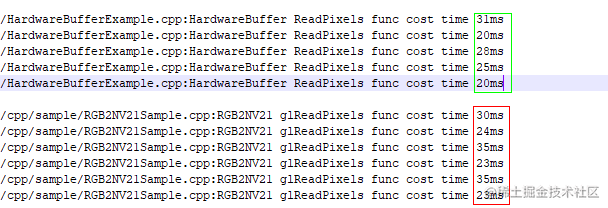Android

Android